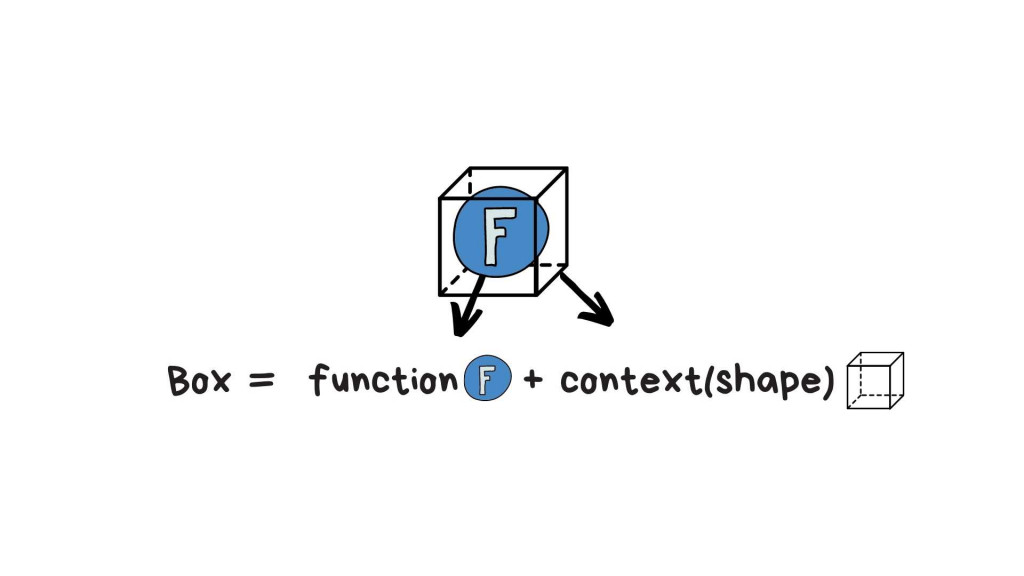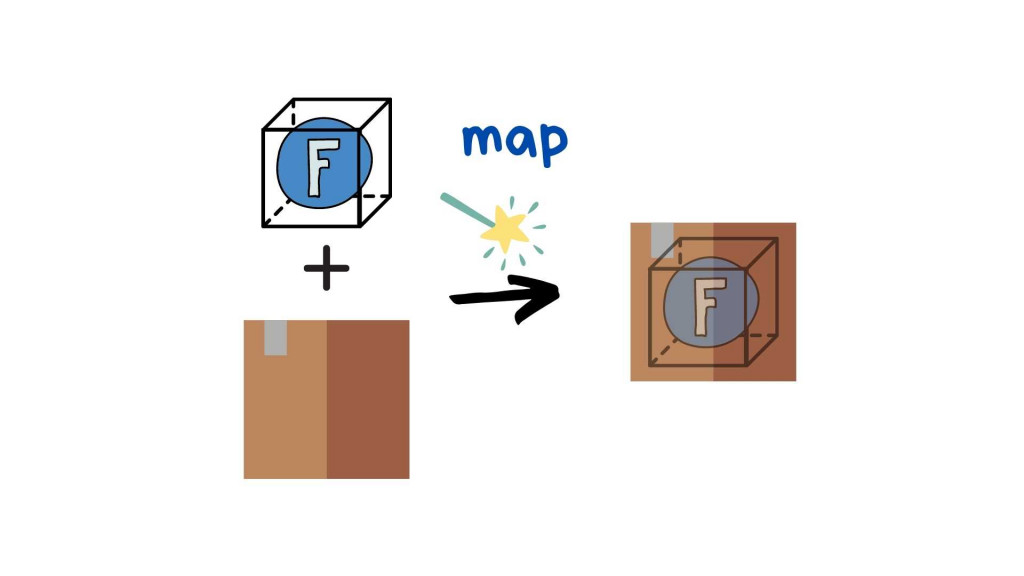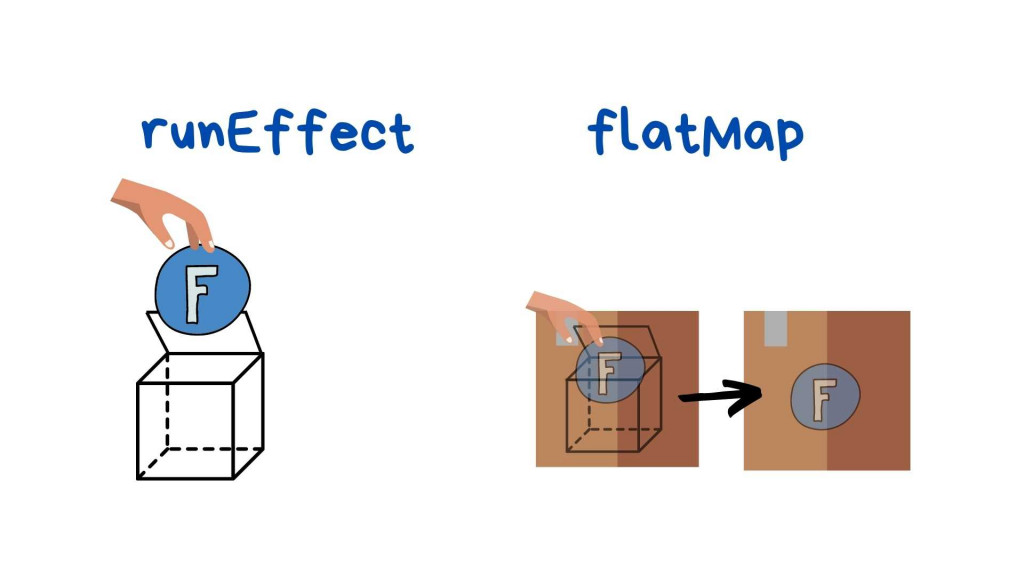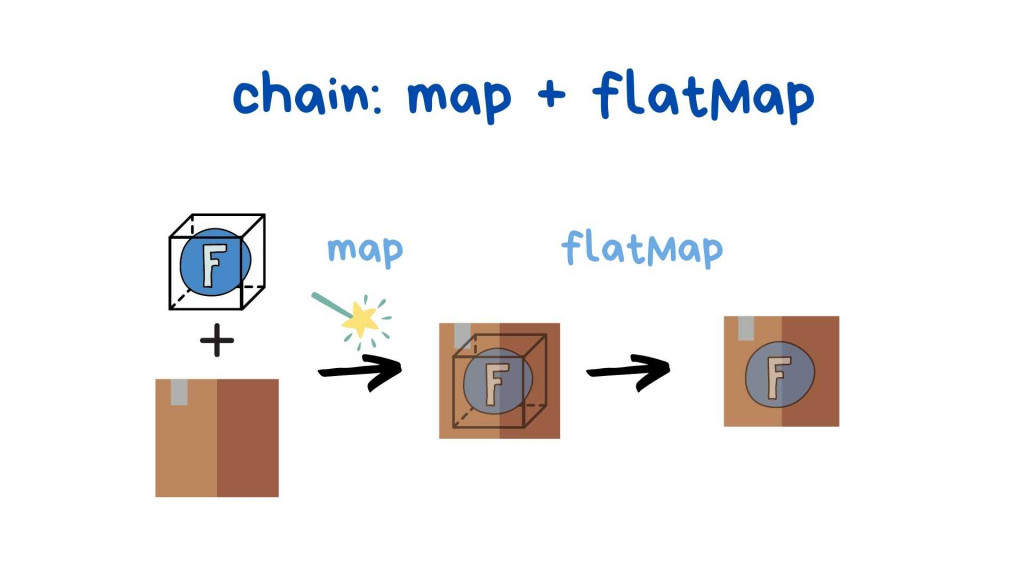#DAY 24
0
Software Development

## Functor 4: 圖解 Box Data Type 之方法 map、flatMap、chain

Functor 對初學者來說實在有點難懂，所以不知不覺已來到第四篇，希望看完這一系列可以真的懂他在幹嘛。 基本上除了系列 1 之外，其他都是在探究 Box 這種 data type(↑ 不知道已出現幾次的 Box data type 圖)

## Map``````const Box = f => ({
map: g => Box( x => g(f(x)) ),
}
``````

## FlatMap

un-nest 扁平化，接受 Functor 當作參數，回傳把最外層 Context 拿掉後裡面的東西``````const Box = f => ({
flatMap: x =>  f(x)
}
``````

``````const Box = f => ({
flatMap: x =>  f(x)，
runEffect: x=> f(x)
}
``````

## Chain

map + flatMap``````const Box = f => ({
map: g => Effect( x => g(f(x)) ),
flatMap: x =>  f(x),
chain: g =>  Effect(f).map(g).flatMap()
}
``````

``````如有錯誤或需要改進的地方，拜託跟我說。

``````